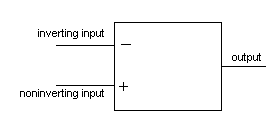Home > Electronic Tutorials > General Theory > Difference Amplifier Tutorial

General Theory - Electronic Tutorials

# Difference Amplifier Tutorial

• The difference amplifier has two inputs and one output.
• It amplifies the difference between the voltages at the two inputs.
• If the voltage on one input is 10 mV and 15 mV on the other then the difference is 5 mV.
• If the amplifier amplifies by ten times then the output voltage will 5 mV times 10 which equals 50 mV.If the two inputs are joined together and a voltage applied to them, then the voltage on both inputs will be the same. There is no difference between them and there will be no output from the amplifier.

Even if the input voltage is varied there will be no output.

If, when being used as a difference amplifier, there is some interference picked up by both inputs, the interfering signal will not appear at the output because both input signals are the same. Only a difference in inputs will produce an output.